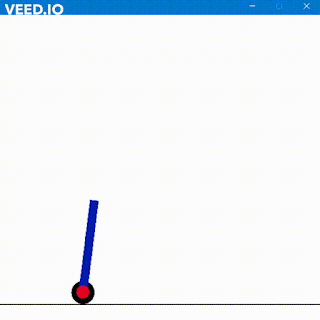### Deep Copy and Shallow Copy

System Verilog Shallow Copy and Deep Copy

Shallow Copy

Whenever we create a variable for instantiating a class a name is created and then when new command is called then an object gets created. However assigning a new handle name with a prior handle will point to the same handle until new command has been executed.
Example
Parent p; will create a variable named p which has to handle the object from the class Parent.
p = new; or p = new(); will create an object of the class Parent. Creating another variable named q and assigning p to it will point to the same object p.
Parent p = new;
Parent q ;
q = p;
Here q and p will point to the same object. q here is just the copy of the object p with same pointing.
Now creating a new object for q as q = new; and then assigning p to q by passing q = p; command will copy contents of p in q but will point to different objects.
Follow this example for better understanding
class Parent; //Parent Class
integer x;
function over();
\$display("This is x%d",x);
endfunction:over
endclass:Parent

module mainclass;
initial begin
Parent p = new;
Parent q = p;
p.x = 10;
p.over();
q.over();
end
endmodule

This will give output :Both object currently point to object p hence same output.
Now change the line Parent q = p; to this Parent q = new; and output will beNow Parent q = new ; creates a new object thus points to a different location and x hasn’t been assigned for object q i.e. we haven’t assigned q.x value here that is why we are getting x as output.
Adding another line q = p; after Parent q = new; This will copy the object p to q. This is what we call shallow copy.
Output will be like this:Adding another line q.x = 12; and remove the line q = p; we are assigning a different value inside object q. Now both p and q are independent and point to different locations. So setting q = p; makes them dependent so avoiding it will make them independent.
Output:Shallow copy will copy every variable across including handles excluding objects. So when we change the value of any member from one object it gets modified in the other object as well.
If we run
q.x = q.x + 1; (Here q.x was 10 initially)
Thus will set x in object q to 11 and will also set x in object p to 11;
Run this code
class Parent; //Parent Class
integer x;
function over();
\$display("This is x%d",x);
endfunction:over
endclass:Parent

module mainclass;
initial begin
Parent p = new;
Parent q = new;
q = p;
p.x = 10;
p.over();
q.over();
q.x = q.x + 1;
q.over();
p.over();
end

Output for thisFurther changing q.x = 65536; we get a same behavior.This happens because at every time q = p; is working here so any change will modify both. The point comes that how can we change in each object independently with copying also so the other object doesn’t get affected. Then instead of assigning q = p; we will modify Parent q = new; to this line Parent q = new p;
Now if we execute this code.
class Parent; //Parent Class
integer x;
function over();
\$display("This is x%d",x);
endfunction:over
endclass:Parent

module mainclass;
initial begin
Parent p = new;
Parent q = new p;
p.x = 10;
q.x = 12;
p.over();
q.over();
q.x = 65536;

q.over();
p.over();
end
endmodule
We will get this output-Thus both objects are now independent. So q = x; made it dependent and same pointer and q = new p; will make both of them independent.

Deep Copy
For deep copy we have a syntax .copy() which is a user made task in the class. Deep copy copies variable and fields including the objects. Any change in one will not change in the other after a deep copy.
Running this example will make it clear
class Parent; //Parent Class
integer mar;
this.mar = par.mar;
endclass:Parent

module mainclass;
initial begin
Parent p = new;
Parent q = new;
p.mar = 10;
q.copy(p);
\$display("After copy p object %d After copy q object %d",p.mar,q.mar);
q.mar = q.mar + 10;
p.mar = p.mar + 30;
\$display("Modified p object %d Modified q object %d",p.mar,q.mar);
end
endmodule

The task copy will copy every variable value into the class member by this command. Then we can increment or decrement both object members independently.
Here is the outputShare and Subscribe :)